3 篇文章 0 订阅

# Introduction

2019年3月更新：最近略忙，不，是超忙，更的会慢些，我先把《神经网络与深度学习》翻译完。

# Preliminaries

## Cumulative Distribution Function (CDF)，累计分布函数

F X ( x ) = P ( X ≤ x ) F_X(x) = P(X \le x)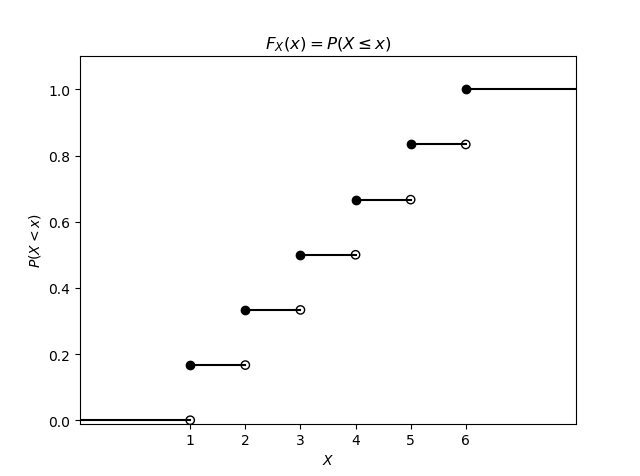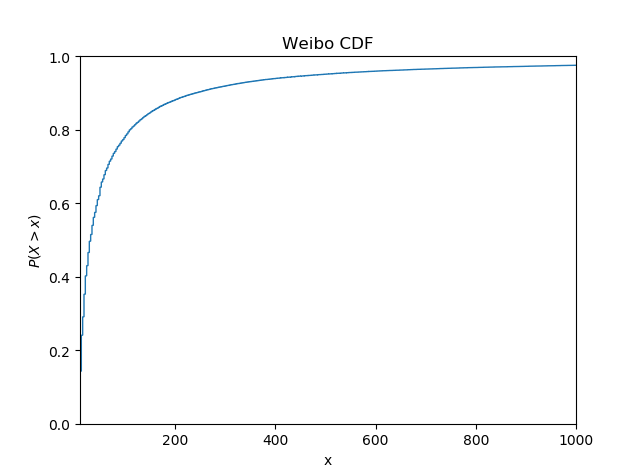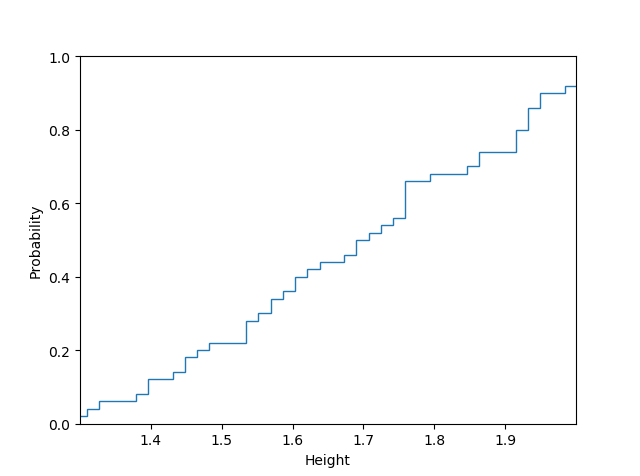### Complementary Cumulative Distribution Function (CCDF)，互补累积分布函数

F ˉ X ( x ) = P ( X > x ) = 1 − F X ( x ) \bar{F}_X(x) = P(X > x) = 1 - F_X(x)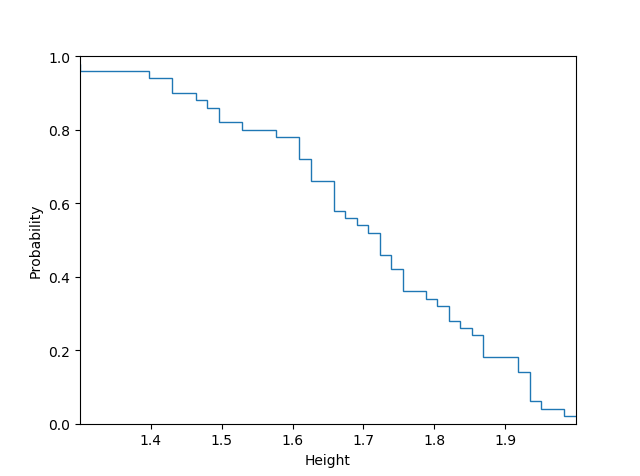## Quantile Funtion (PPF)，分位函数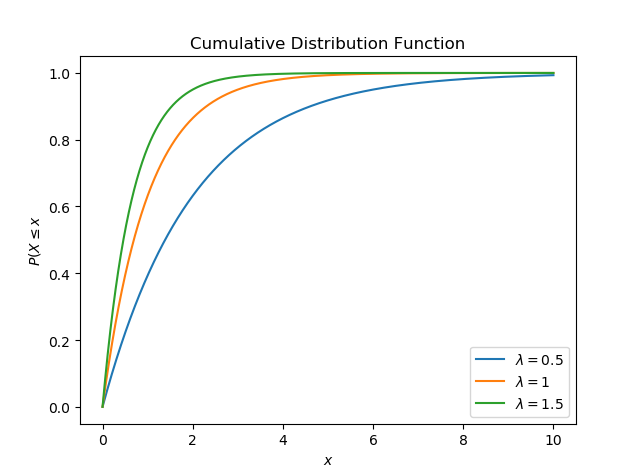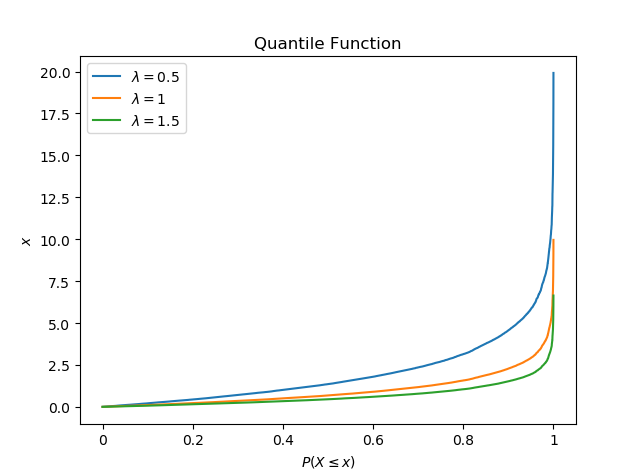## Exponential Distribution，指数分布

f ( x ; λ ) = { λ e − λ x x ≥ 0 , 0 x < 0. f(x;\lambda) = \begin{cases} \lambda e^{-\lambda x} & x \ge 0, \\ 0 & x < 0. \end{cases}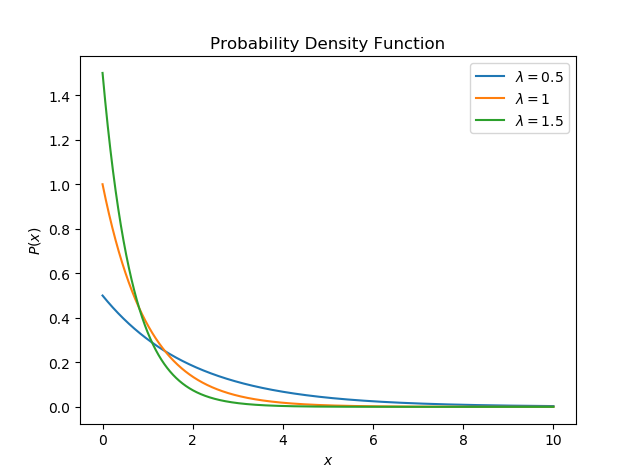λ \lambda 越小表示单位时间内事件发生的次数越少。由图可知，随着 x x 的增加，事件发生的概率越来越小。

F ( x ; λ ) = { 1 − e − λ x x ≥ 0 , 0 x < 0. F(x;\lambda) = \begin{cases} 1 - e^{-\lambda x} & x \ge 0, \\ 0 & x < 0. \end{cases}## Heavy-tailed Distribution 重尾分布

lim ⁡ x → ∞ e t x Pr [ X > x ] = ∞           for all  t > 0 \lim_{x \rarr \infty} e^{tx} \text{Pr}[X > x] = \infty \;\;\;\; \text{ for all } t > 0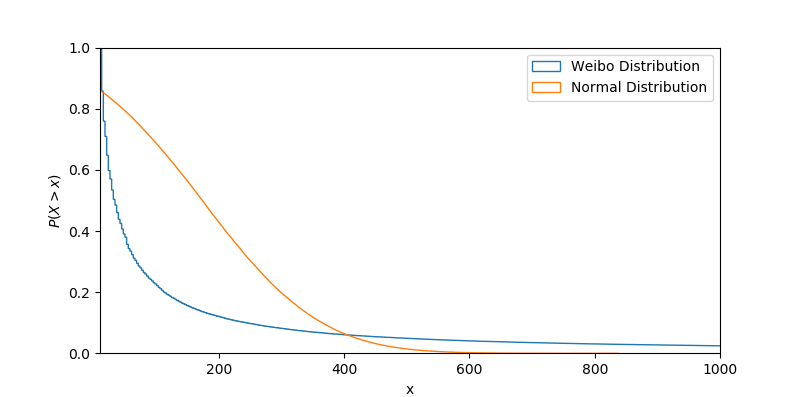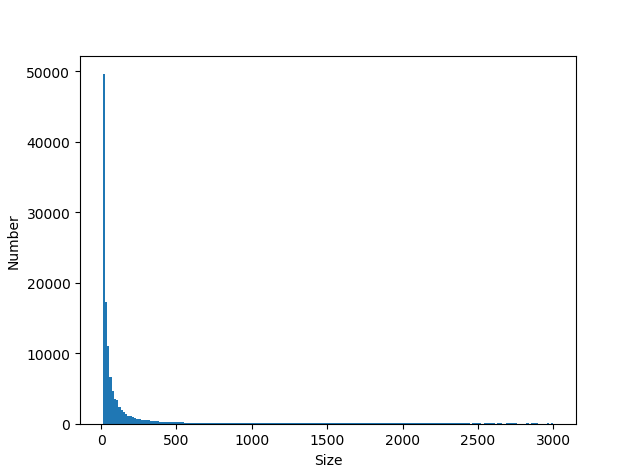### Long-tailed Distribution，长尾分布

lim ⁡ x → ∞ Pr [ X > x + t ∣ X > x ] = 1 \lim_{x \rarr \infty} \text{Pr} [X > x + t | X > x] = 1
Pr [ X > x ] \text{Pr}[X > x] 就是我们前面说过的CCDF。长尾分布与重尾分布相似但不同，长尾分布都是重尾分布，但重尾分布不一定是长尾分布。微博数据集虽然符合重尾分布，但是，根据常识我们知道，一条微博被转发1,000次和被转发2,000次的概率是不一样的，显然有
lim ⁡ x → ∞ Pr [ X > 2000 ∣ X > 1000 ] < 1 \lim_{x \rarr \infty} \text{Pr} [X > 2000 | X > 1000] < 1

### Subexponential Distribution，次指数分布

Pr [ X 1 + X 2 ≤ x ] = F ∗ 2 ( x ) = ∫ − ∞ ∞ F ( x − y ) d F ( y ) . \text{Pr}[X_1 + X_2 \le x] = F^{*2}(x) = \int_{-\infty}^{\infty} F(x - y)dF(y).

F ∗ n ‾ ( x ) ∼ n F ‾ ( x )          as  x → ∞ \overline{F^{*n}} (x) \sim n \overline{F}(x) \;\;\;\; \text{as }x \rarr \infty

Pr [ X 1 + X 2 + ⋯ + X n > x ] ∼ Pr [ m a x ( X 1 , X 2 , … , X n ) ]          x → ∞ \text{Pr}[X_1+ X_2 + \dots + X_n > x] \sim \text{Pr}[max(X_1, X_2, \dots, X_n)] \;\;\;\; x \rarr \infty

### Fat-tailed Distribution，肥尾分布

Pr [ X > x ] ∼ x − α          as  x → ∞ ,          α > 0 \text{Pr} [X > x] \sim x^{-\alpha} \;\;\;\; \text{as } x \rarr \infty, \;\;\;\; \alpha > 0
α \alpha 不很大的时候，如果一个分布满足上述条件(即CCDF等价 x − α x^{-\alpha} ），则它可以称为肥尾分布。

## Pareto Distribution

CDF公式投喂：
F ‾ ( x ) = Pr [ X > x ] = { 1 − ( x m x ) a x ≥ x m , 0 x < x m . \overline{F}(x) = \text{Pr}[X > x] = \begin{cases} 1-(\frac {x_m}{x})^a & x \ge x_m, \\ 0 & x < x_m. \end{cases}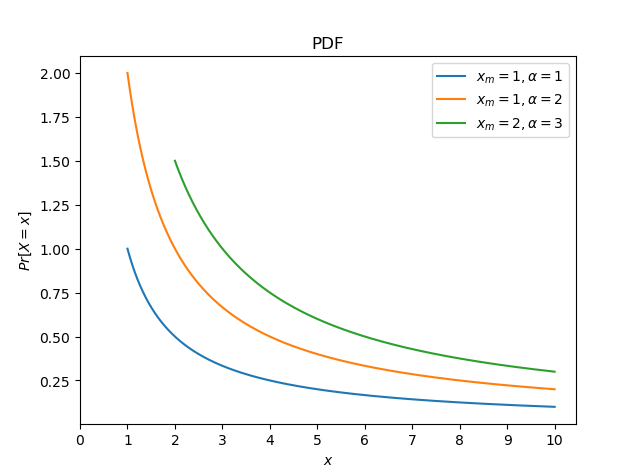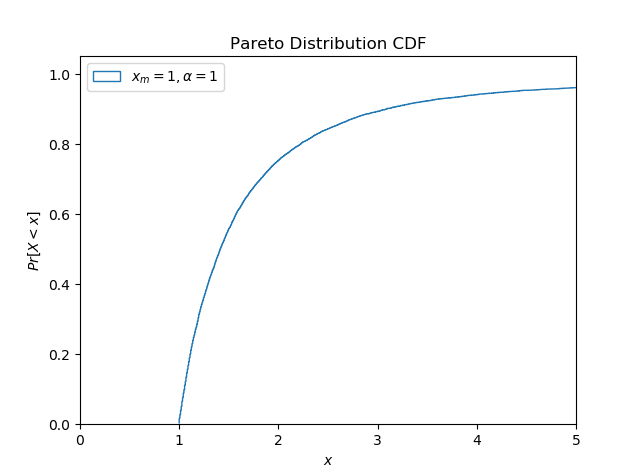# Code

## Cumulative Distribution Function

mu = 1.7
sigma = 0.2
n_bins = 50

np.random.seed(3197747)
height = np.random.normal(mu, sigma, n_bins)

plt.hist(height, n_bins, density=True, histtype='step', cumulative=True)

plt.axis([1.3, 2.0, 0, 1])
plt.xticks([1.4, 1.5, 1.6, 1.7, 1.8, 1.9])
plt.xlabel('Height')
plt.ylabel('Probability')

plt.show()


## Quantile Funtion

lmbda_list = [0.5, 1, 1.5]
x = 1 - np.random.random(10000)

fig, ax = plt.subplots()

for lmbda in lmbda_list:
# y = [(1 - np.e ** (-1 * lmbda * x_)) for x_ in x[:]]
y = [-math.log(x_)/lmbda for x_ in x]
label = '$\lambda =$' + str(lmbda)
ax.plot(sorted(y), label=label)

plt.title('Quantile Function')

plt.xticks(np.arange(0, 10001, 2000), ('0', '0.2', '0.4', '0.6', '0.8',
'1'))
plt.xlabel('$P(X \leq x)$')
plt.ylabel('$x$')
plt.legend()
plt.show()


## Exponential Distribution Function

# Probability Density Function
lmbda_list = [0.5, 1, 1.5]
x = np.arange(0, 10, 0.001)

fig, ax = plt.subplots()

for lmbda in lmbda_list:
y = [(lmbda * np.e ** (-1 * lmbda * x_)) for x_ in x[:]]
label = '$\lambda =$' + str(lmbda)
ax.plot(x, y, label=label)

plt.title('Probability Density Function')
plt.xlabel('$x$')
plt.ylabel('$P(x)$')
plt.legend()
plt.show()

# CDF
lmbda_list = [0.5, 1, 1.5]
x = np.arange(0, 12, 0.001)

fig, ax = plt.subplots()

for lmbda in lmbda_list:
y = [(1 - np.e ** (-1 * lmbda * x_)) for x_ in x[:]]
label = '$\lambda =$' + str(lmbda)
ax.plot(x, y, label=label)

plt.title('Cumulative Distribution Function')
plt.xlabel('$x$')
plt.ylabel('$P(X \leq x$')
plt.legend()
plt.show()


## Pareto Distribution

# PDF
fig, ax = plt.subplots()

alpha = [1, 2, 3]
x_m = [1, 1, 2]
for i in range(len(alpha)):
x = np.arange(x_m[i], 10, 0.001)
y = [(alpha[i]*pow(x_, alpha[i])/(pow(x_, alpha[i]+1))) for x_ in x]
label = '$x_m = ' + str(x_m[i]) + ' ,\\alpha =$' + str(alpha[i])
ax.plot(x, y, label=label)

plt.xlabel('$x$')
plt.ylabel('$Pr[X = x]$')
plt.title('PDF')
plt.xticks(np.arange(0, 11))
plt.legend()
plt.show()

# CDF
fig, ax = plt.subplots()

alpha = [1, 2, 3]
x_m = [1, 1, 2]
for i in range(len(alpha)):
np.random.seed(3197747)
x = 1 - np.random.random(10000)
# reverse function
y = [(alpha[i] * pow(x_m[i], alpha[i]) / x_) ** (1 / (alpha[i] + 1))
for x_ in x]
label = '$x_m = ' + str(x_m[i]) + ' ,\\alpha =$' + str(alpha[i])

ax.hist(y, len(y), cumulative=True, density=True, histtype='step',
label=label)

plt.xlim(0, 5)
plt.xlabel('$x$')
plt.ylabel('$Pr[X < x]$')
plt.title('Pareto Distribution CDF')
plt.legend(loc=2)
plt.show()


# Reference

6. 林建希. (2007). 关于次指数分布及其相关类的一个性质. Journal of Xiamen University (Nature Science), Retrieved from http://www.doc88.com/p-1963143584484.html
9. dymodi. (January 8, 2017). 重尾分布，长尾分布，肥尾分布 和 随机游走 （Heavy-tailed, Long-tailed, Fat-tailed distribution and Random walk）. Retrieved from https://blog.csdn.net/dymodi/article/details/54231728
12. Cao, Q., Shen, H., Cen, K., Ouyang, W., & Cheng, X. (2017, November). DeepHawkes: Bridging the gap between prediction and understanding of information cascades. In Proceedings of the 2017 ACM on Conference on Information and Knowledge Management (pp. 1149-1158). ACM.
12-31
05-092万+09-146983
10-022145
08-279646
11-242768
05-212万+
06-103万+
07-193万+
05-316813
01-142215
03-061万+
09-263636
07-23741
09-053万+
02-272498
11-08844
10-273930
04-23319点击重新获取扫码支付余额充值